# Pythagorean theorem - 9th grade (14y) - math problems

#### Number of problems found: 525

• Equilateral triangle v2Equilateral triangle has a perimeter 36 dm. What is its area?
• 4s pyramidRegular tetrahedral pyramid has a base edge a=17 and collaterally edge length b=32. What is its height?
• KostkaKostka je vepsána do koule o poloměru r = 6 cm. Kolik procent tvoří objem kostky z objemu koule?
• Square circles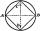Calculate the length of the described and inscribed circle to the square ABCD with a side of 5cm.
• Park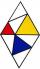In the park is marked diamond shaped line connecting locations A, D, S, C, B, A. Calculate its length if |AB| = 108 m, |AC| = 172.8 m.
• TetrahedronCalculate height and volume of a regular tetrahedron whose edge has a length 4 cm.
• Distance between 2 pointsFind the distance between the points (7, -9), (-1, -9)
• Equilateral triangleCalculate the area of an equilateral triangle with circumference 72cm.
• Equilateral triangleThe equilateral triangle has a 23 cm long side. Calculate its content area.
• TriangleCalculate the sides of the triangle if its area S = 630 and the second cathethus is shorter by 17.
• Right angleIf a, b and c are two sides of a triangle ABC, a right angle in A, find the value on each missing side. If b=10, c=6
• Body diagonalFind the cube surface if its body diagonal has a size of 6 cm.
• Triangular pyramidWhat is the volume of a regular triangular pyramid with a side 3 cm long?
• Semicircle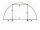To a semicircle with diameter 10 cm inscribe square. What is the length of square sides?
• Is right triangleDecide if the triangle XYZ is rectangular: x = 4 m, y = 6 m, z = 4 m
• Vertices of RTShow that the points P1 (5,0), P2 (2,1) & P3 (4,7) are the vertices of a right triangle.
• Hexagon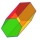Calculate the surface of a regular hexagonal prism whose base edge a = 12cm and side edge b = 3 dm.
• WindbreakA tree at a height of 3 meters broke in the windbreak. Its peak fell 4.5 m from the tree. How tall was the tree?
• Find diagonal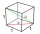Find the length of the diagonal of a cuboid with length=20m width=25m height=150m
• EQL triangle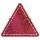Calculate inradius and circumradius of equilateral triangle with side a=77 cm.

Do you have an interesting mathematical word problem that you can't solve it? Submit a math problem, and we can try to solve it.

We will send a solution to your e-mail address. Solved examples are also published here. Please enter the e-mail correctly and check whether you don't have a full mailbox.

Please do not submit problems from current active competitions such as Mathematical Olympiad, correspondence seminars etc...

Pythagorean theorem is the base for the right triangle calculator.